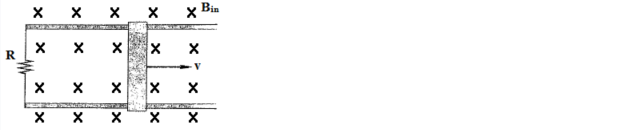# Problem: A metal bar is pulled to the right perpendicular to a uniform magnetic field. The bar rides on parallel metal rails connected through a resistor, as shown in the figure below, so the apparatus makes complete circuit. Find the direction of the current induced in the circuit in two ways: a) By looking at the magnetic force on the charges in the moving bar. b) Using Lenz's Law

###### FREE Expert Solution
90% (431 ratings)
###### Problem Details

A metal bar is pulled to the right perpendicular to a uniform magnetic field. The bar rides on parallel metal rails connected through a resistor, as shown in the figure below, so the apparatus makes complete circuit. Find the direction of the current induced in the circuit in two ways:

a) By looking at the magnetic force on the charges in the moving bar.

b) Using Lenz's LawFrequently Asked Questions

What scientific concept do you need to know in order to solve this problem?

Our tutors have indicated that to solve this problem you will need to apply the Motional EMF concept. You can view video lessons to learn Motional EMF. Or if you need more Motional EMF practice, you can also practice Motional EMF practice problems.

How long does this problem take to solve?

Our expert Physics tutor, Juan took 4 minutes undefined to solve this problem. You can follow their steps in the video explanation above.

What professor is this problem relevant for?

Based on our data, we think this problem is relevant for Professor Voronine's class at USF.